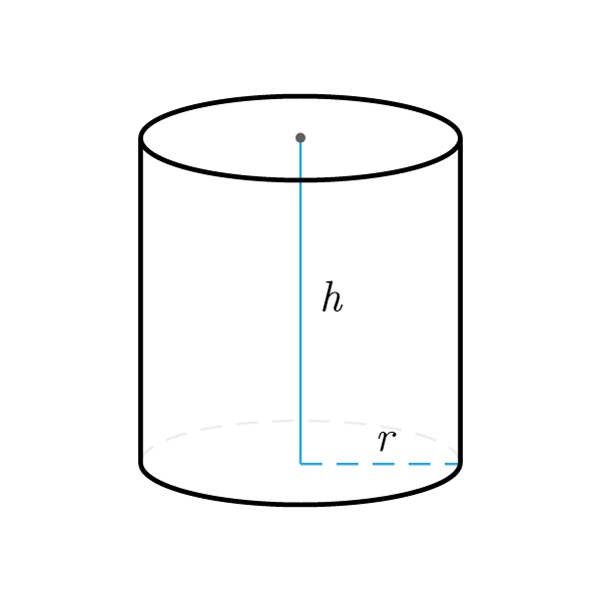# Cylinder

 Radius $$r$$ Height $$h$$
$$V = \pi r \times h$$
Volume
$$r = \sqrt{\frac{V}{\pi h}}$$
$$h = \frac{V}{\pi {r}^2}$$
$$V = A_{base} \times h$$
Volume
$$A_{base} = \frac{V}{h}$$
Base area
$$h = \frac{V}{A_{base}}$$
Height
$$S_{lat} = 2 \pi r \times h$$
Lateral surface
$$r = \frac{V}{2 \pi h}$$
$$h = \frac{V}{2 \pi r}$$
Height
$$A_{base} =\pi {r}^2$$
Base area (Area of ​​the circle)
$$r = \sqrt{\frac{A_{base}}{\pi}}$$
$$S_{tot} = 2 \times A_{base} + S_{lat}$$
Total surface
$$S_{lat} = S_{tot} - 2 \times A_{base}$$
Lateral surface
$$A_{base} = \frac{S_{tot} - S_{lat}}{2}$$
Base area
Equilateral Cylinder
$$2r = h$$
Diameter

## Definition

The cylinder is a solid obtained by the complete rotation of a rectangle around one of its sides.

## Properties

1. In the rectangle that generates the cylinder, the side around which the rotation occurs is the height of the cylinder, while the other side is the radius.
2. The height of the cylinder is generally represented by a segment that joins the centers of the two bases, more generally the height of the cylinder is any perpendicular segment that joins the two bases.

## Other definitions

• An equilateral cylinder is a cylinder in which the base diameter and height are congruent.Cylinder Formulas
Data Formula
Volume V = π r × h
Radius r = √( V / (π × h) )
Height h = V / (π × r2)
Volume V = Abase × h
Base area Abase = V / h
Height h = V / Abase
Lateral surface Slat = 2 π r × h
Radius r = V / (2 π h)
Height h = V / (2 π r)
Base area Abase = π r2
Radius r = √( Abase / π )
Total surface Stot = 2 × Abase + Slat
Lateral surface Slat = Stot - 2 × Abase
Base area Abase = (Stot - Slat) / 2
Equilateral Cylinder Formulas
Data Formula
Diameter 2r = h